# Friction and centripetal force.

Satvik Pandey

## Homework Statement

A car goes on a horizontal circular road of radius ##R##, the speed increasing at a constant rate ##\frac{dv}{dt}=a##. The friction coefficient between the road and the tier is ##\mu##. Find the speed at which the car will skid.

## The Attempt at a Solution

I solved it earlier using the fact that only force acting on the system is the friction. So ##F_{net}=Ma_{net}##.
So ##f==m\sqrt { { a }^{ 2 }+\frac { { v }^{ 4 } }{ { R }^{ 2 } } } ##

And the car will slip when ##f=\mu mg##

Using these two equations I got the right answer.

But I am confused now.According to my solution it seems that a component of friction force is providing the tangential acceleration. But friction always opposes the motion then how could it accelerate a body. I mean, it should always decelerate the body.

I have one more confusion.It is well known that friction provides the required centripetal force to move a car in circle. But friction always always opposes the motion and in a small time the car moves in a tangential direction. So friction should act in tangential direction (opposite to the motion of the car). Why does it acts in radially inward direction.

In the above problem it is clear that a component of friction acts in tangential direction. But when a car moves in circle with a constant speed then friction acts in radial direction only. As the car moves in tangential direction in a small period of time so friction should act in tangential direction to oppose the motion of the car.

## Answers and Replies

Homework Helper
According to my solution it seems that a component of friction force is providing the tangential acceleration. But friction always opposes the motion then how could it accelerate a body. I mean, it should always decelerate the body.
Well, it does oppose the motion when friction is used to accelerate something. A wheel would spin with the contact area moving backwards if there were no friction. So we use friction (and Newton 3) to bring about motion too.

Note that the only external force in the horizontal plane acting on the system is friction; the engine delivers torque to drive the wheels.

Same thing for the centripetal force: car would move in a straight line if there were no friction to keep it on a circular trajectory. Racing cars actually skid through turns.

Another issue: there can be friction if there's no motion. Then the friction doesn't do any work. Like a block at rest on a slope. Or a car in a circular trajectory: friction in a radial direction but no motion in that direction.

Satvik Pandey
Well, it does oppose the motion when friction is used to accelerate something. A wheel would spin with the contact area moving backwards if there were no friction. So we use friction (and Newton 3) to bring about motion too.

When the spinning wheel is kept on the ground then friction acts to oppose the spin. Suppose if a wheels roll on a rough surface (also assume a external force is applied at the center) then friction (##f_{s}##) acts on opposite direction to ##F## to satisfy the condition ##a=R \alpha##. But then it acts in backward direction.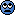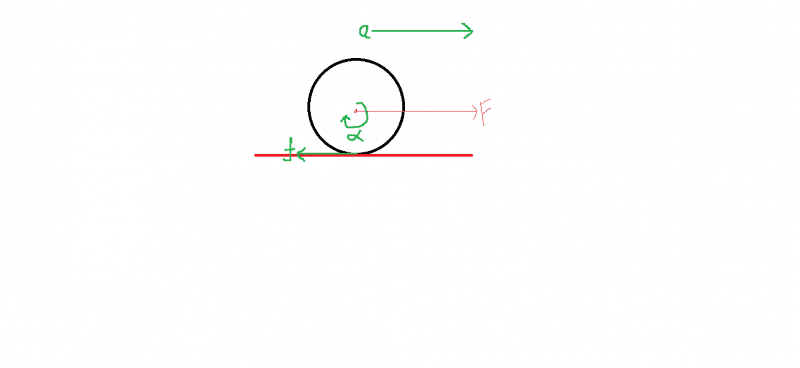Same thing for the centripetal force: car would move in a straight line if there were no friction to keep it on a circular trajectory. Racing cars actually skid through turns.
I was wondering, why friction acts in the tangential direction only when the car is accelerating? I mean, even if it moves in a circle with constant speed then it's tangential motion should be opposed.
I thought about that and I came up with this--
If we assume assume that car's wheel rolls on the road then if it moving with constant speed then no external force should act in tangential direction. As the wheel is rolling so static friction should act in tangential direction but as no other force is acting on it so frictional force would be zero (as it is a static friction so it can be zero). But in radial direction ##mv^{2}/r## is acting so ##f_{s}=mv^{2}/r## (assuming that, the car does not slips). What do think BvU, is my explanation correct?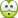Homework Helper
When the spinning wheel is kept on the ground then friction acts to oppose the spin. Suppose if a wheels roll on a rough surface (also assume a external force is applied at the center) then friction (f s f_{s} ) acts on opposite direction to F F to satisfy the condition a=Rα a=R \alpha . But then it acts in backward direction.This is not what was described in the problem statement ! Where, tell me, does this mysterious red F come from ? Is there a horse pulling the car or something ? In that case yes, the friction to make the wheel rotate is pointing backwards.

But running, cycling, skating, accelerating a car (or having pulled by a horse) is done by pushing backwards and then the friction pushes forward !

Easy to grasp if you imagine the total absence of friction: your feet, bike wheel, skate, car wheel, horse hooves, etc. would shoot backwards.

But in the case you describe in post #3, with total absence of friction, the wheel would slide forward without turning: there's nothing that exercises a torque.

•Satvik Pandey
Homework Helper
Look at it this way: there's useful friction that helps you accelerate and brake, let's call it 'grip'. We want it as big as possible.

And there's nuisance friction such as wind resistance, rolling resistance, etc. We want it as small as possible. So we shape cars like bananas, use ball bearings on the axles, pump up our tyres, and so on.

I was wondering, why friction acts in the tangential direction only when the car is accelerating? I mean, even if it moves in a circle with constant speed then it's tangential motion should be opposed. -- hard to comment; I don't follow what you mean with "it moves in a circle with constant speed then it's tangential motion should be opposed"

I thought about that and I came up with this--
If we assume assume that car's wheel rolls on the road then if it moving with constant speed then no external force should act in tangential direction.
-- actually, you need to compensate for wind resistance by pushing the road backwards: sum of external forces is zero

As the wheel is rolling so static friction should act in tangential direction but as no other force is acting on it so frictional force would be zero (as it is a static friction so it can be zero). -- where the wheel touches the road, the rubber is moving straight down, then up again. Hence the desire for hard tyres and a hard road surface: that minimizes nuisance resistance. So that kind of resistance isn't all that big.

But in radial direction mv 2 /r mv^{2}/r is acting so f s =mv 2 /r f_{s}=mv^{2}/r (assuming that, the car does not slips). -- you make it seem as if that's two things, when it is only one: FS. (But I may be wrong).

What do think BvU, is my explanation correct?-- try to split the problem a bit: some things are more easily considered when thinking about linear motion.

Satvik Pandey
This is not what was described in the problem statement ! Where, tell me, does this mysterious red F come from ? Is there a horse pulling the car or something ? In that case yes, the friction to make the wheel rotate is pointing backwards.

But running, cycling, skating, accelerating a car (or having pulled by a horse) is done by pushing backwards and then the friction pushes forward !

Easy to grasp if you imagine the total absence of friction: your feet, bike wheel, skate, car wheel, horse hooves, etc. would shoot backwards.

But in the case you describe in post #3, with total absence of friction, the wheel would slide forward without turning: there's nothing that exercises a torque.

So, the engine of the vehicle actually rotates the wheels by providing a torque and then friction which acts at the base of the wheels, accelerates the vehicle in forward direction. Right?
Look at it this way: there's useful friction that helps you accelerate and brake, let's call it 'grip'. We want it as big as possible.

And there's nuisance friction such as wind resistance, rolling resistance, etc. We want it as small as possible. So we shape cars like bananas, use ball bearings on the axles, pump up our tyres, and so on.

I meant that , when a car moves in circle with a constant velocity then the acceleration of car is ##v^{2}/r## as only external force acting on the system is friction so ##f=mv^{2}/r##. This means friction in such case acts radially inward. But in a small time it moves linearly.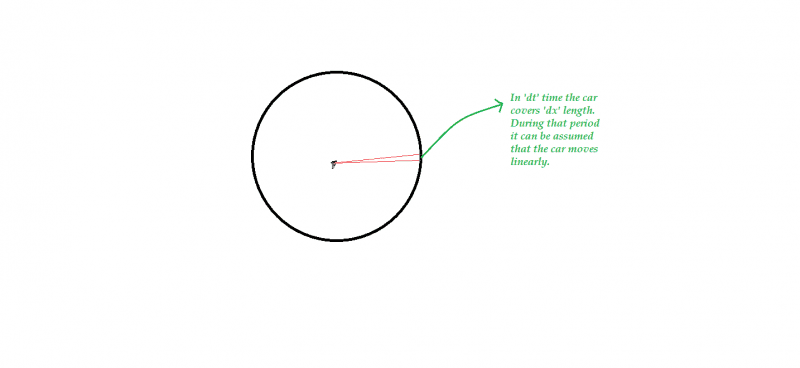Then why friction does not act in tangential direction. However, when the car have tangential acceleration then friction acts in tangential direction also.
I mean that when a car moves with constant velocity in a circle then no friction acts along the tangent but when the same car moves in circle with tangential acceleration then friction acts along the tangent. Why this happens?

My explanation--
If we assume assume that car's wheel rolls on the road then if it moving with constant speed (in a circle) then no external force should act in tangential direction.(assuming negligible air resistance).
As the wheel is rolling so static friction should act in tangential direction but as no other external force is acting on it so frictional force would be zero (as it is a static friction so it can be zero). So no friction acts in tangential direction.

Homework Helper
So, the engine of the vehicle actually rotates the wheels by providing a torque and then friction which acts at the base of the wheels, accelerates the vehicle in forward direction. Right?
Yes.

I meant that , when a car moves in circle with a constant velocity then the acceleration of car is ##v^{2}/r## as only external force acting on the system is friction so ##f=mv^{2}/r##. This means friction in such case acts radially inward. But in a small time it moves linearly. View attachment 81329

Then why friction does not act in tangential direction. However, when the car have tangential acceleration then friction acts in tangential direction also.
I mean that when a car moves with constant velocity in a circle then no friction acts along the tangent but when the same car moves in circle with tangential acceleration then friction acts along the tangent. Why this happens?

Friction is a force, it has both tangential and radial component. Even the mechanism as it acts can be different. See the pattern on a tyre.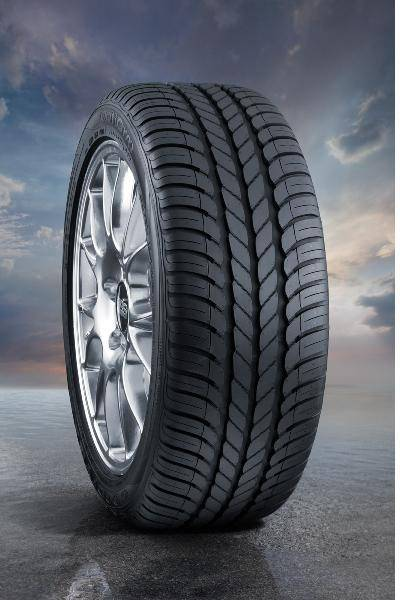The ribs near the rim are a bit like those of a cogwheel. When the tyre accelerates, they push the ground horizontally, and it pushes back providing the accelerating force to the car.

In a curve, the tyre must not slide radially outward, perpendicular to its direction of travel. The static friction acting perpendicularly to the rim prevents that sliding.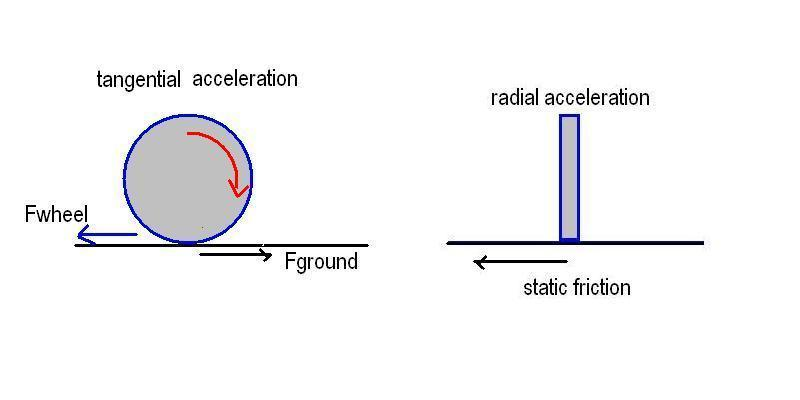Fwheel is the force, the spinning wheel pushes the ground, and Fgound is the force, the ground pushes the car forward.
The friction is static in both cases, it prevents the surfaces in contact from sliding. But the wheel is active in the first case, it exerts force on the ground which pushes back. In the second case, friction opposes centrifugal force (or supplies the centripetal force needed to turning round).

My explanation--
If we assume assume that car's wheel rolls on the road then if it moving with constant speed (in a circle) then no external force should act in tangential direction.(assuming negligible air resistance).
As the wheel is rolling so static friction should act in tangential direction but as no other external force is acting on it so frictional force would be zero (as it is a static friction so it can be zero). So no friction acts in tangential direction.

Yes, (almost no) force acts in tangential direction if the speed is constant.

Last edited:
•Satvik Pandey
Satvik Pandey
In a curve, the tyre must not slide radially outward, perpendicular to its direction of travel. The static friction acting perpendicularly to the rim prevents that sliding.

View attachment 81342

The friction is static in both cases, it prevents the surfaces in contact from sliding. But the wheel is active in the first case, it exerts force on the ground which pushes back. In the second case, friction opposes centrifugal force (or supplies the centripetal force needed to turning round).

Friction (tangential component) acting on the wheels accelerate (supports the motion) the wheels in tangential direction. But in the you diagram(1) it acts in opposite direction of the motion of the wheel.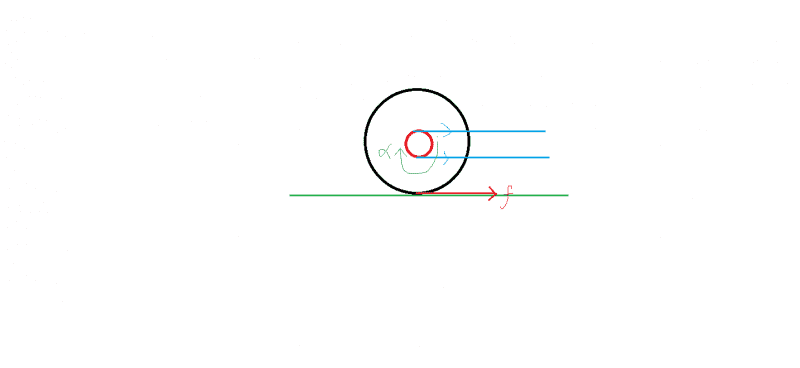The force acting at the gear provides angular acceleration to the wheels.right? So the base of the wheels tends to slip in backward direction. So friction acts in forward direction. right?

As the force acting at the gear are internal force so they can not contribute to the CoM of the vehicle. So. friction acting the rear wheels are responsible for the motion of the vehicle. However the front wheel is not directly connected to the engine so friction acting on that is responsible for providing the the angular acceleration to the wheels so it should act in backward direction (opposite to the motion of the CoM of the vehicle). So net force which is responsible for the motion for the acceleration of the CoM of the vehicle is ##f_{rear wheels}-f_{front wheels}##. Am I right?

Homework Helper
Friction (tangential component) acting on the wheels accelerate (supports the motion) the wheels in tangential direction. But in the you diagram(1) it acts in opposite direction of the motion of the wheel.View attachment 81375
The force acting at the gear provides angular acceleration to the wheels.right? So the base of the wheels tends to slip in backward direction. So friction acts in forward direction. right?

I meant Fground the force the ground acts on the wheel and it is forward. Fwheel is the force, the spinning wheel pushes the ground backward.

As the force acting at the gear are internal force so they can not contribute to the CoM of the vehicle. So. friction acting the rear wheels are responsible for the motion of the vehicle. However the front wheel is not directly connected to the engine so friction acting on that is responsible for providing the the angular acceleration to the wheels so it should act in backward direction (opposite to the motion of the CoM of the vehicle). So net force which is responsible for the motion for the acceleration of the CoM of the vehicle is ##f_{rear wheels}-f_{front wheels}##. Am I right?

You are completely right.
The engine rotates the gear with increasing angular speed and that causes angular acceleration of the connected wheels. The wheels push the ground backwards, the ground pushes the whole car forward, together with the front wheels. If they were not able to rotate, the contact surface of those wheels would move forward. So the friction acts backward there, accelerating their rotation.

•Satvik Pandey
Satvik Pandey
I meant Fground the force the ground acts on the wheel and it is forward. Fwheel is the force, the spinning wheel pushes the ground backward.

You are completely right.
The engine rotates the gear with increasing angular speed and that causes angular acceleration of the connected wheels. The wheels push the ground backwards, the ground pushes the whole car forward, together with the front wheels. If they were not able to rotate, the contact surface of those wheels would move forward. So the friction acts backward there, accelerating their rotation.

Thank you ehild.You are great.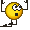Thank you BvU for helping me.